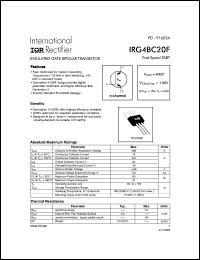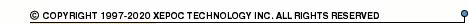More than12 565 012 queries processedIRG4BC20 series datasheets. Manufacturer: International Rectifier.

 IRG4BC20 Insulated gate bipolar transistor. VCES = 600V, VCE(on)typ. = 1.66V @ VGE = 15V, IC = 9.0A in 3-pin TO-220AB package. Operational temperature range from -55°C to 150°C. Datasheet*) IRG4BC20FD-S Insulated gate bipolar transistor with ultrafast soft recovery diode. VCES = 600V, VCE(on)typ. = 1.66V @ VGE = 15V, IC = 9.0A in 3-pin DDPak package. Operational temperature range from -55°C to 150°C. Datasheet*) IRG4BC20FD Insulated gate bipolar transistor with ultrafast soft recovery diode. VCES = 600V, VCE(on)typ. = 1.66V @ VGE = 15V, IC = 9.0A in 3-pin TO-220AB package. Operational temperature range from -55°C to 150°C. Datasheet*) IRG4BC20K-S Insulated gate bipolar transistor. VCES = 600V, VCE(on)typ. = 2.27V @ VGE = 15V, IC = 9.0A in 3-pin DDPak package. Operational temperature range from -55°C to 150°C. Datasheet*) IRG4BC20K Insulated gate bipolar transistor. VCES = 600V, VCE(on)typ. = 2.27V @ VGE = 15V, IC = 9.0A in 3-pin TO-220AB package. Operational temperature range from -55°C to 150°C. Datasheet*) IRG4BC20KD-S Insulated gate bipolar transistor with ultrafast soft recovery diode. VCES = 600V, VCE(on)typ. = 2.27V @ VGE = 15V, IC = 9.0A in 3-pin DDPak package. Operational temperature range from -55°C to 150°C. Datasheet*) IRG4BC20KD Insulated gate bipolar transistor with ultrafast soft recovery diode. VCES = 600V, VCE(on)typ. = 2.27V @ VGE = 15V, IC = 9.0A in 3-pin TO-220AB package. Operational temperature range from -55°C to 150°C. Datasheet*) IRG4BC20MD-S Insulated gate bipolar transistor with ultrafast soft recovery diode. VCES = 600V, VCE(on)typ. = 1.85V @ VGE = 15V, IC = 11A in 3-pin DDPak package. Operational temperature range from -55°C to 150°C. Datasheet*) IRG4BC20MD Insulated gate bipolar transistor with ultrafast soft recovery diode. VCES = 600V, VCE(on)typ. = 1.85V @ VGE = 15V, IC = 11A in 3-pin TO-220AB package. Operational temperature range from -55°C to 150°C. Datasheet*) IRG4BC20S Insulated gate bipolar transistor. VCES = 600V, VCE(on)typ. = 1.4V @ VGE = 15V, IC = 10A in 3-pin TO-220AB package. Operational temperature range from -55°C to 150°C. Datasheet*) IRG4BC20SD-S Insulated gate bipolar transistor with ultrafast soft recovery diode. VCES = 600V, VCE(on)typ. = 1.4V @ VGE = 15V, IC = 10A in 3-pin DDPak package. Operational temperature range from -55°C to 150°C. Datasheet*) IRG4BC20SD Insulated gate bipolar transistor with ultrafast soft recovery diode. VCES = 600V, VCE(on)typ. = 1.4V @ VGE = 15V, IC = 10A in 3-pin TO-220AB package. Operational temperature range from -55°C to 150°C. Datasheet*) IRG4BC20U Insulated gate bipolar transistor. VCES = 600V, VCE(on)typ. = 1.85V @ VGE = 15V, IC = 6.5A in 3-pin TO-220AB package. Operational temperature range from -55°C to 150°C. Datasheet*) IRG4BC20UD-S Insulated gate bipolar transistor with ultrafast soft recovery diode. VCES = 600V, VCE(on)typ. = 1.85V @ VGE = 15V, IC = 6.5A in 3-pin DDPak package. Operational temperature range from -55°C to 150°C. Datasheet*) IRG4BC20UD Insulated gate bipolar transistor with ultrafast soft recovery diode. VCES = 600V, VCE(on)typ. = 1.85V @ VGE = 15V, IC = 6.5A in 3-pin TO-220AB package. Operational temperature range from -55°C to 150°C. Datasheet*) IRG4BC20W-S Insulated gate bipolar transistor. VCES = 600V, VCE(on)typ. = 2.16V @ VGE = 15V, IC = 6.5A in 3-pin DDPak package. Operational temperature range from -55°C to 150°C. Datasheet*) IRG4BC20W Insulated gate bipolar transistor. VCES = 600V, VCE(on)typ. = 2.16V @ VGE = 15V, IC = 6.5A in 3-pin TO-220AB package. Operational temperature range from -55°C to 150°C. Datasheet*) IRG4BC20F INSULATED GATE BIPOLAR TRANSISTOR(Vces=600V, Vce(on)typ.=1.66V, @Vge=15V, Ic=9.0A) Datasheet*) IRG4BC20FD-STRL INSULATED GATE BIPOLAR TRANSISTOR WITH ULTRAFAST SOFT RECOVERY DIODE(Vces=600V, Vce(on)typ.=1.66V, @Vge=15V, Ic=9.0A) Datasheet*) IRG4BC20KS INSULATED GATE BIPOLAR TRANSISTOR(Vces=600V, Vce(on)typ.=2.27V, @Vge=15V, Ic=9.0A) Datasheet*) IRG4BC20MDS INSULATED GATE BIPOLAR TRANSISTOR WITH ULTRAFAST SOFT RECOVERY DIODE(Vces=600V, Vce(on)typ.=1.85V, @Vge=15V, Ic=11A) Datasheet*) IRG4BC20SDS INSULATED GATE BIPOLAR TRANSISTOR WITH ULTRAFAST SOFT RECOVERY DIODE(Vces=600V, Vce(on)typ.=1.4V, @Vge=15V, Ic=10A) Datasheet*) IRG4BC20UDS INSULATED GATE BIPOLAR TRANSISTOR WITH ULTRAFAST SOFT RECOVERY DIODE(Vces=600V, Vce(on)typ.=1.85V, @Vge=15V, Ic=6.5A) Datasheet*) IRG4BC20WS INSULATED GATE BIPOLAR TRANSISTOR(Vces=600V, Vce(on)typ.=2.16V, @Vge=15V, Ic=6.5A) Datasheet*)